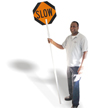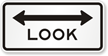# Traffic Signs Quiz- Advanced Quiz

## Can you answer these 17 questions?

1.

Where is this sign used?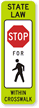2.

What does this sign mean?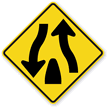3.

What does this sign mean?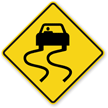4.

What does this sign mean?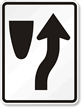5.

What does this sign mean?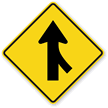6.

What does this sign mean?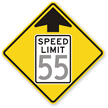7.

What does this sign mean?8.

What does this sign mean?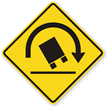9.

Where is this sign used?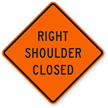10.

What does this sign mean?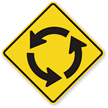11.

What does this sign mean?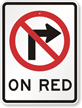12.

What does this sign mean?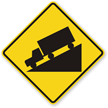13.

Where is this sign used?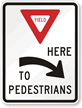14.

What does this sign mean?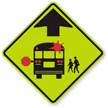15.

What does this sign mean?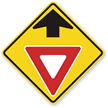16.

Where is this sign used?